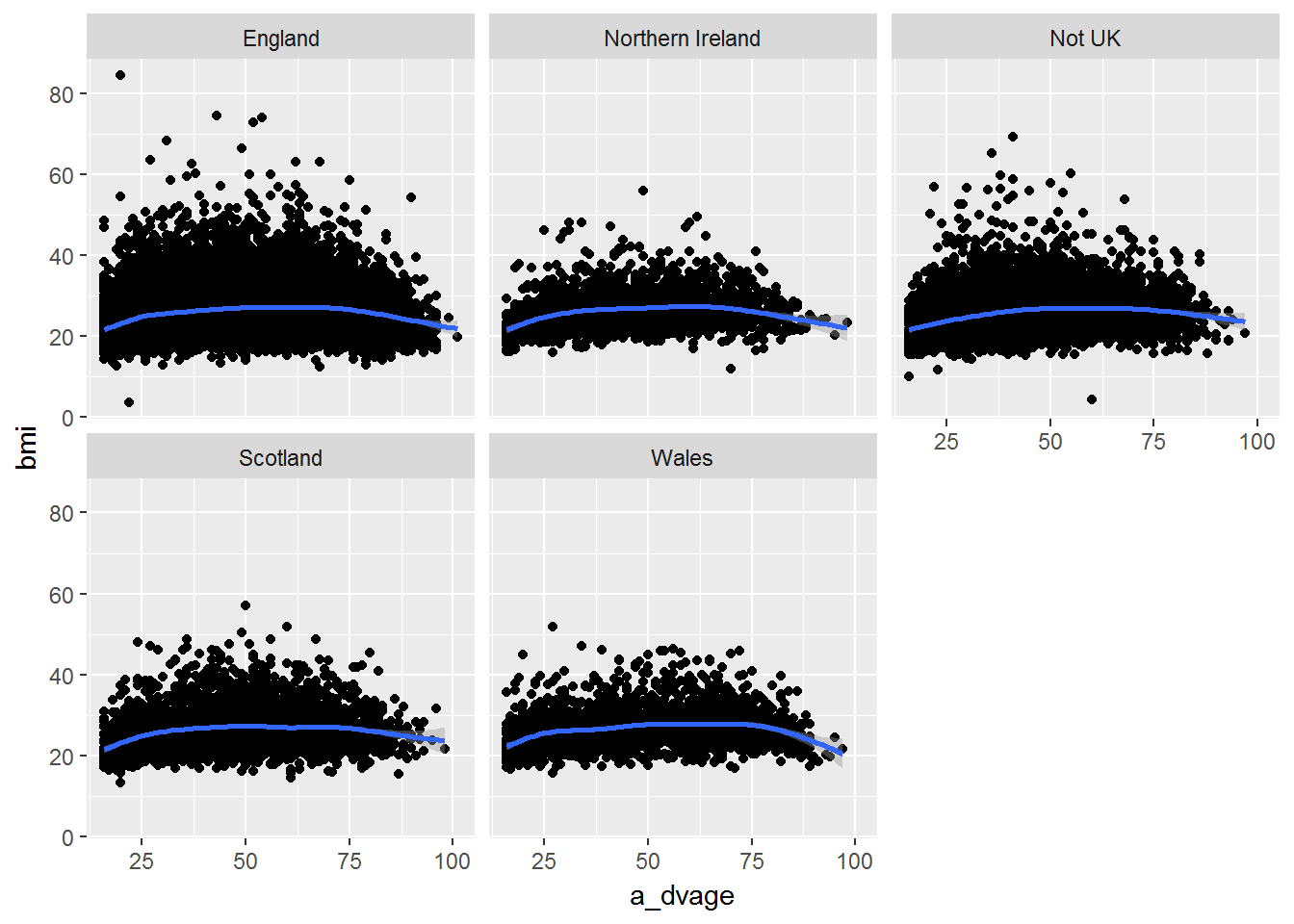# 7 Data visualisation

Pre-requisite for this class: ch.3 (“Data visualisation”) from R for Data Science - http://r4ds.had.co.nz/data-visualisation.html

At home you learned about the basic principles of data visualisation in R with the ggplot2 package. Let us see how we can apply this to the Understanding Society data set.

Personally I can never remember all the details of the ggplot2 syntax. I often use the ready-made “recipes” from the R Graphics Cookbook by W.Chang – https://www.amazon.co.uk/R-Graphics-Cookbook-Winston-Chang/dp/1449316956/. The 2nd edition is coming out later this year – https://www.amazon.co.uk/Graphics-Cookbook-2e-Winston-Chang/dp/1491978600 .

You may also find Winston Chang’s website useful (and not only for graphics) - http://www.cookbook-r.com .

## 7.1 Reading in the data

First let us read in the data we used in week 2 when we learned about dplyr (a short version of the wave 1 data) and recreate the measures for weight, height and BMI.

library(tidyverse)
library(data.table)
head(W1, 3)
## # A tibble: 3 x 15
##       pidp a_sex a_dvage a_ukborn a_hlht a_hlhtf a_hlhti a_hlhtc a_hlwt
##      <int> <int>   <int>    <int>  <int>   <int>   <int>   <int>  <int>
## 1 68001367     1      39        1      1       6       0      -8      1
## 2 68004087     1      59        5      1       5      11      -8      2
## 3 68006127     2      39        1      1       5       1      -8      1
## # ... with 6 more variables: a_hlwts <int>, a_hlwtp <int>, a_hlwtk <int>,
## #   heightcm <dbl>, weightkg <dbl>, bmi <dbl>

## 7.2 Visualising one quantitative variable

Exercise. Visualise the distribution of the BMI with ggplot2. Which statistical graphs would be appropriate for this?

### 7.2.1 Histogram.

ggplot(W1, aes(x=bmi)) +
geom_histogram(bins = 100) +
xlab("Body mass index")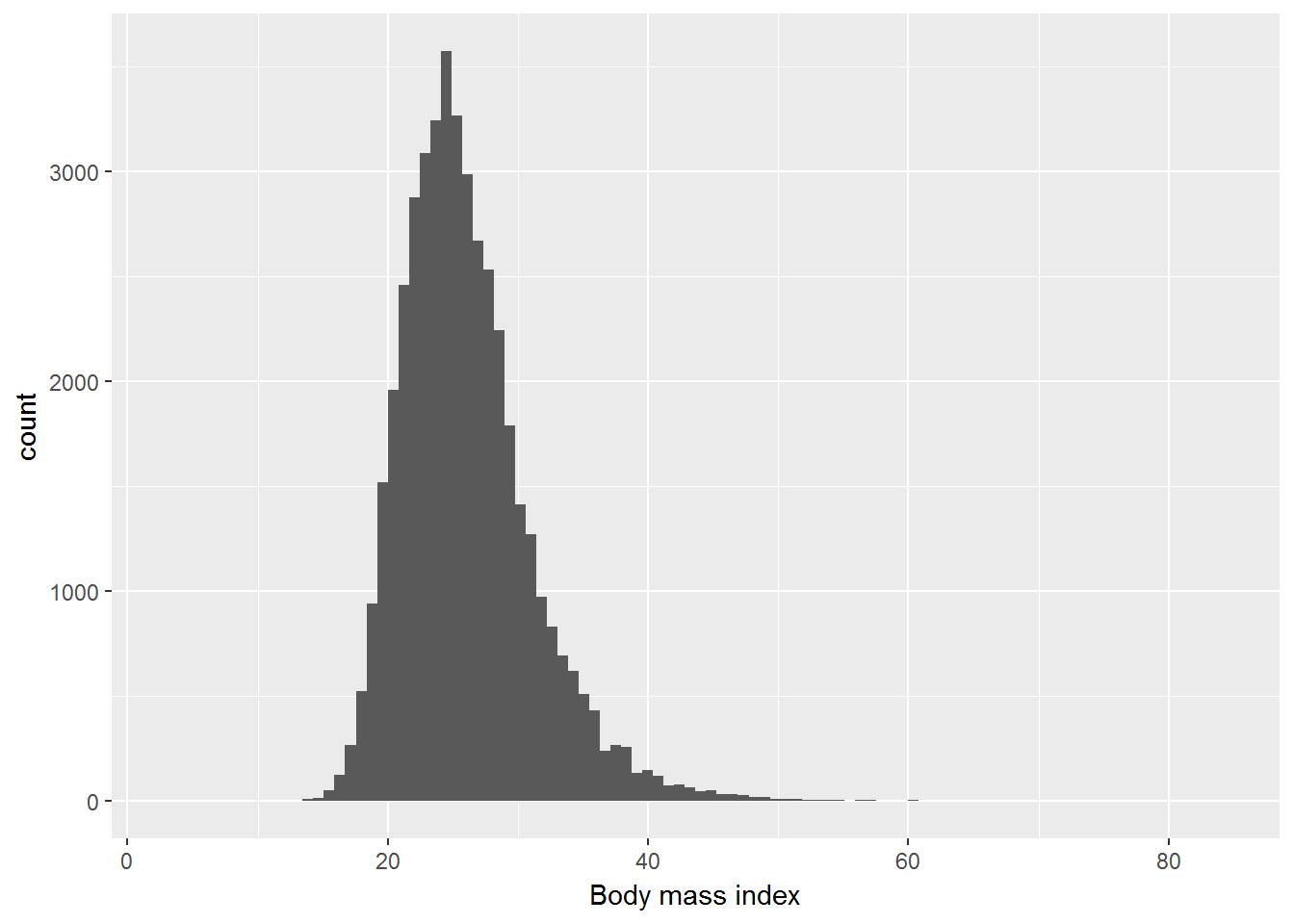### 7.2.2 Density chart.

ggplot(W1, aes(x=bmi)) +
geom_density() +
xlab("Body mass index")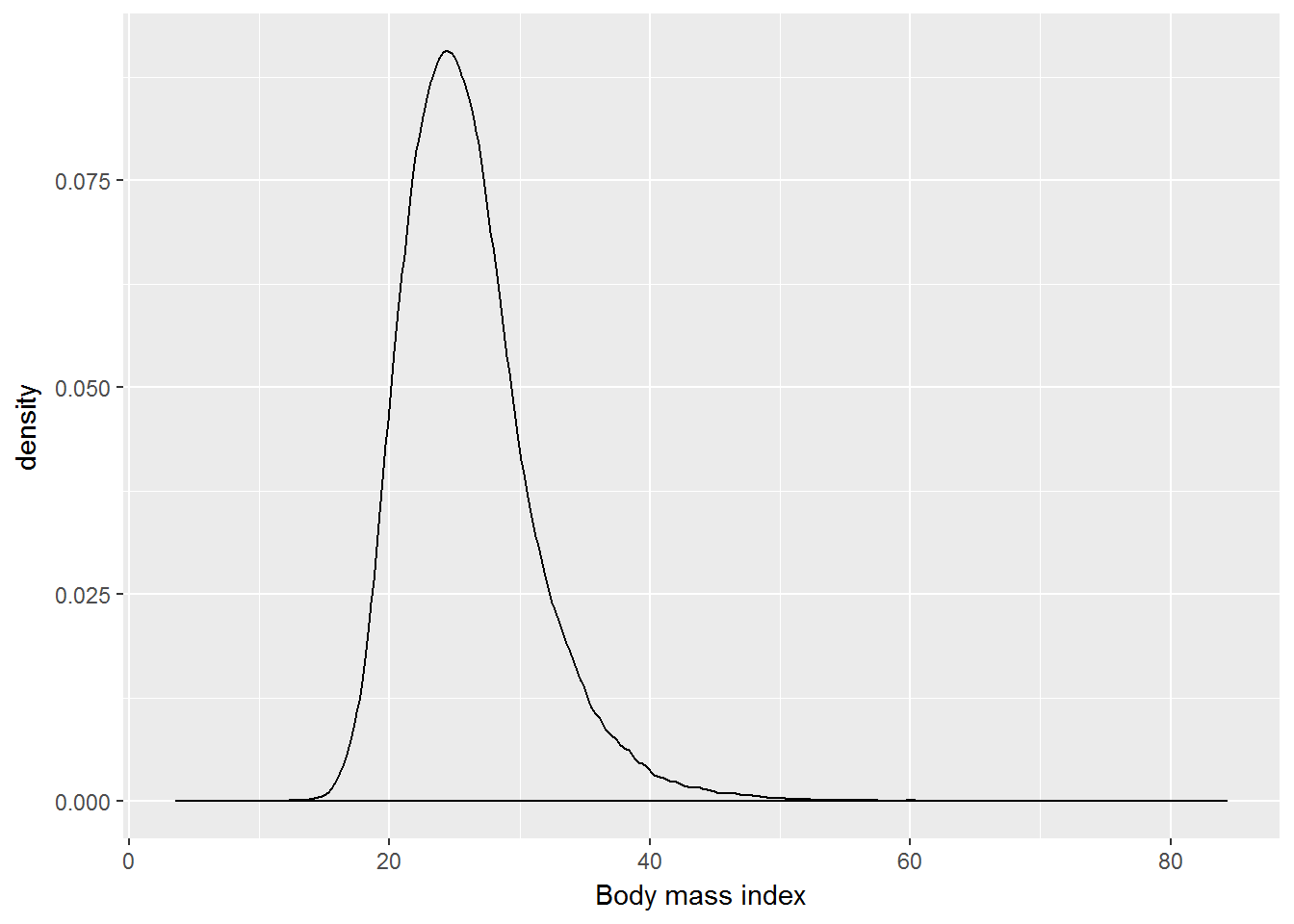## 7.3 Visualising one categorical variable

Exercise. Visualise the distribution of a_ukborn with ggplot2. Which statistical graphs would be appropriate for this?

### 7.3.1 Bar plot.

table(W1$a_ukborn) ## ## -9 -2 -1 1 2 3 4 5 ## 6 2 8 33480 3567 2154 2033 9744 W1 <- W1 %>% mutate(a_ukborn = ifelse(a_ukborn > 0, a_ukborn, NA)) %>% mutate(cbirth = recode(a_ukborn, "1" = "England", "2" = "Scotland", "3" = "Wales", "4" = "Northern Ireland", "5" = "Not UK")) table(W1$cbirth)
##
##          England Northern Ireland           Not UK         Scotland
##            33480             2033             9744             3567
##            Wales
##             2154
W1 %>%
filter(!is.na(cbirth)) %>%
ggplot(aes(x=cbirth)) +
geom_bar() +
xlab("Country of birth")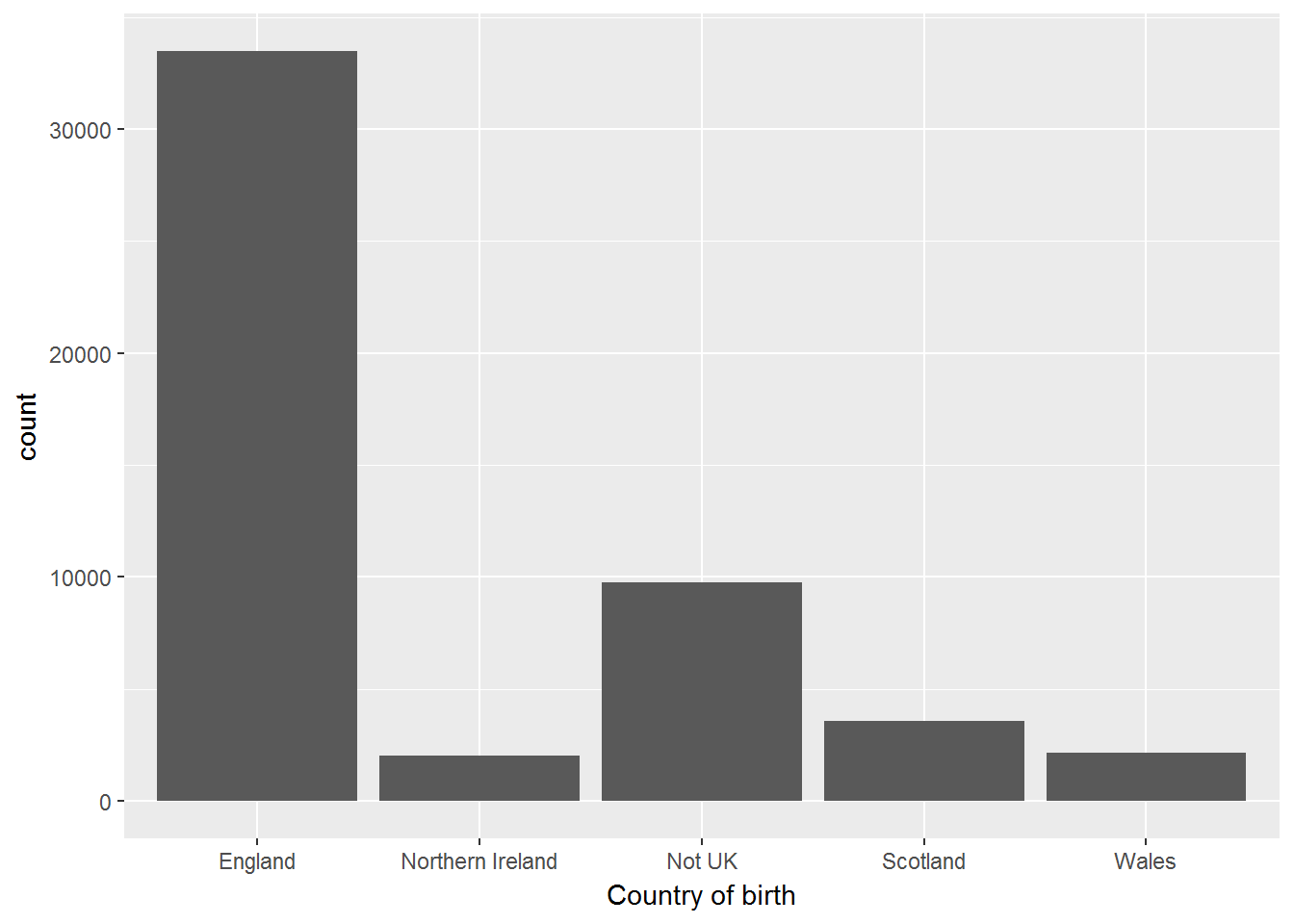table(W1$cbirth, useNA = "always") ## ## England Northern Ireland Not UK Scotland ## 33480 2033 9744 3567 ## Wales <NA> ## 2154 16 ## 7.4 Visualising two quantitative variables Exercise. Visualise the joint distribution of weight (in kg) and height (in cm). In your chart show the regression line and the nonparametric smoothing line. ggplot(W1, aes(x = weightkg, y= heightcm)) + geom_point() + geom_smooth() + stat_smooth(method=lm)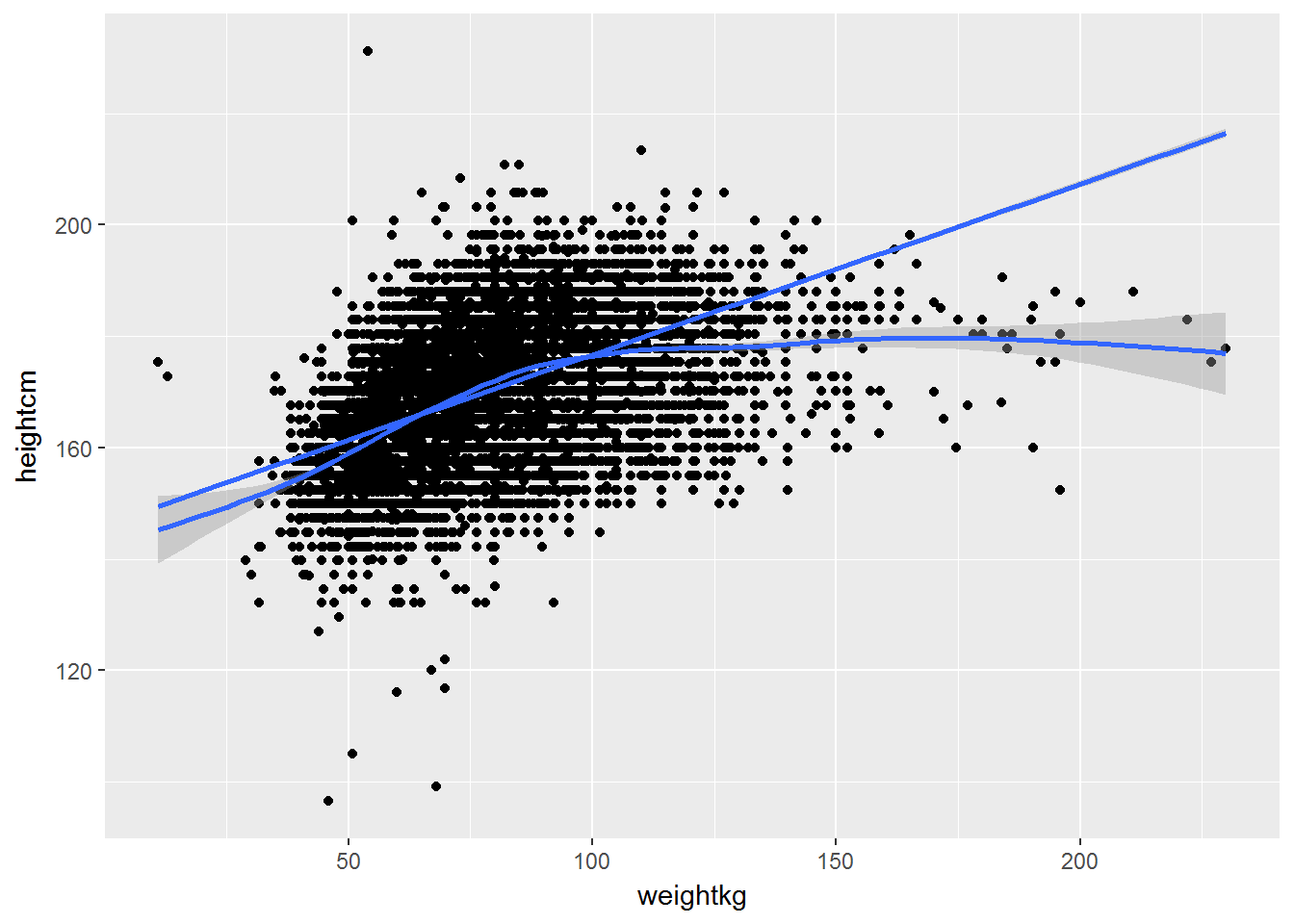## 7.5 Visualising one categorical and one quantitative variable Exercise. Visualise the distribution of BMI for a) men and women, b) different age groups. # Coding a categorical variable for age groups table(W1$a_dvage, useNA = "always")
##
##   16   17   18   19   20   21   22   23   24   25   26   27   28   29   30
##  937  864  787  798  786  738  756  786  806  791  827  849  878  936  914
##   31   32   33   34   35   36   37   38   39   40   41   42   43   44   45
##  884  879  917  864  928  983  923  976 1051 1054 1032 1043  935  968  987
##   46   47   48   49   50   51   52   53   54   55   56   57   58   59   60
##  940  941  917  889  873  824  817  765  803  722  761  703  756  666  662
##   61   62   63   64   65   66   67   68   69   70   71   72   73   74   75
##  820  806  775  685  621  646  591  521  563  571  500  498  443  411  380
##   76   77   78   79   80   81   82   83   84   85   86   87   88   89   90
##  368  356  338  294  287  267  225  207  166  147  132  108   80   73   54
##   91   92   93   94   95   96   97   98   99  100  101 <NA>
##   38   27   20   21   14   14    4    3    2    1    1    0
W1 <- W1 %>%
mutate(agegr = ifelse(a_dvage < 31, "16-30",
ifelse(a_dvage > 30 & a_dvage < 46, "31-45",
ifelse(a_dvage > 45 & a_dvage < 61, "46-60",
">60")))) %>%
mutate(agegr = factor(agegr, c("16-30", "31-45", "46-60", ">60")))

ggplot(W1, aes(x = agegr, y= bmi)) +
geom_boxplot() +
xlab("Age group") +
ylab("Body mass index")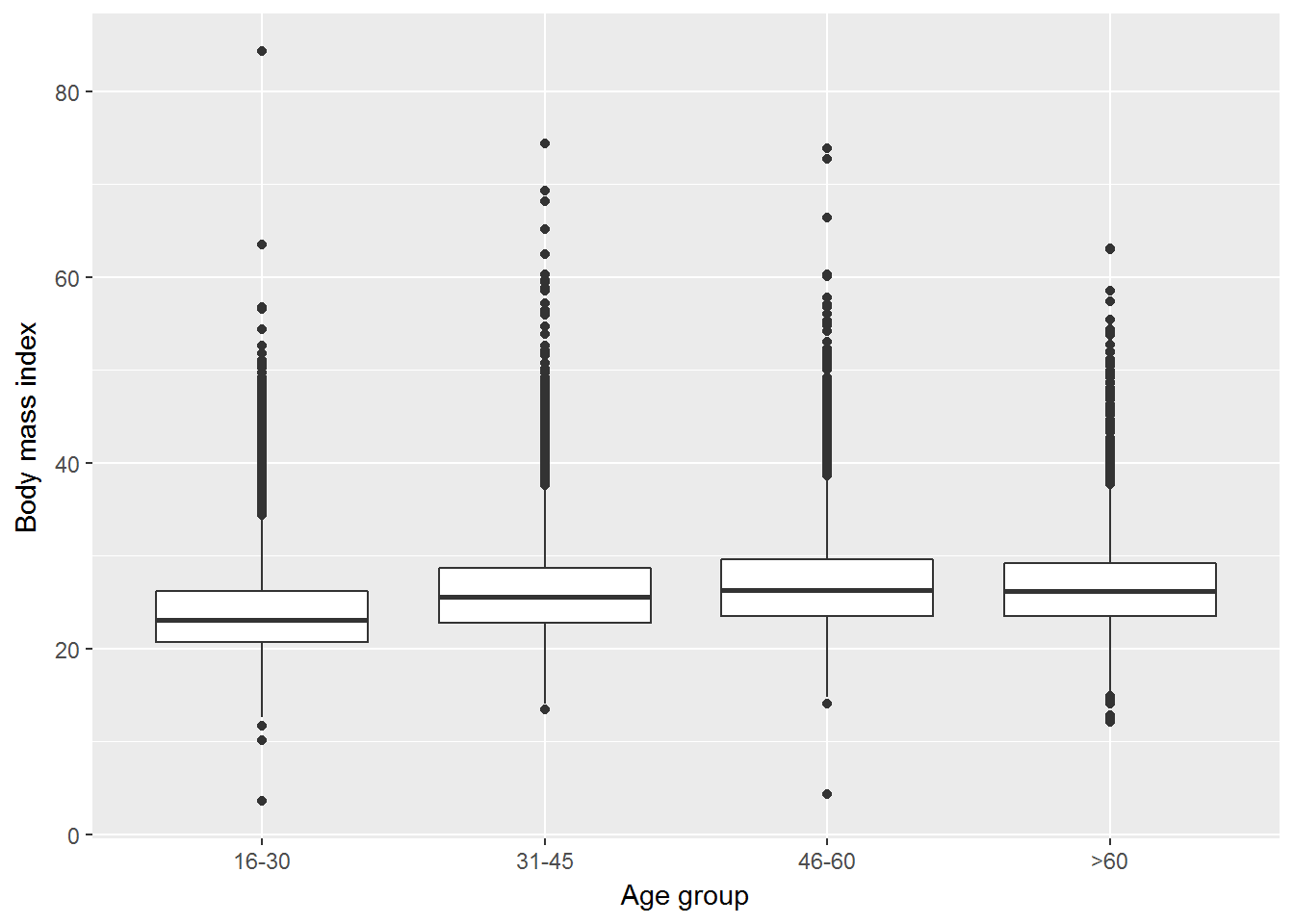## 7.6 Visualising two categorical variables

Exercise. Use facets to visualise the distribution of a_ukborn by age group.

W1 %>%
filter(!is.na(cbirth)) %>%
ggplot(aes(x=cbirth)) +
geom_bar() +
xlab("Country of birth") +
facet_wrap(~ agegr)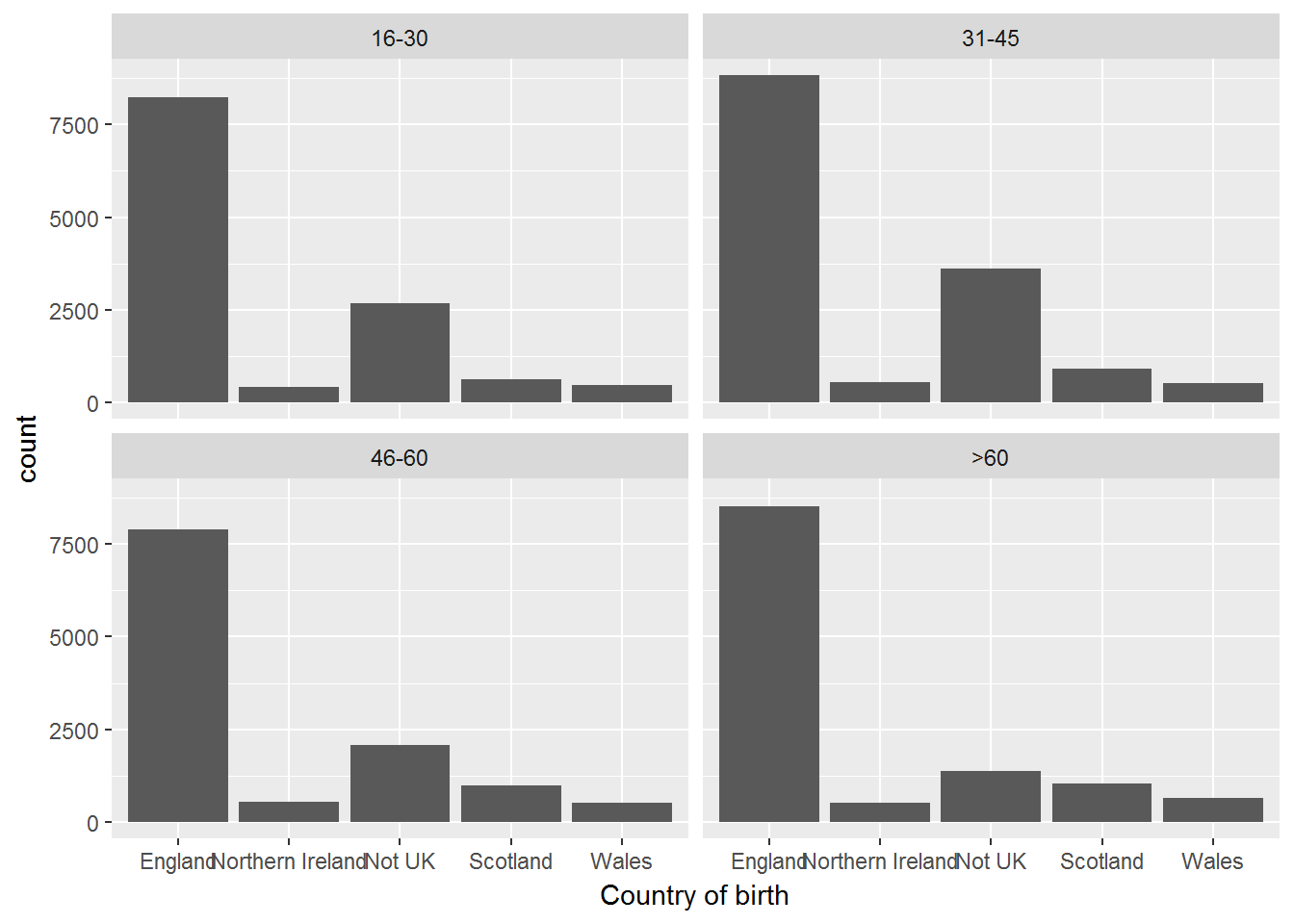Alternatively you can do a jitter plot, but in our case it wouldn’t look nice.

W1 %>%
filter(!is.na(cbirth)) %>%
ggplot(aes(x=cbirth, y = agegr)) +
geom_jitter() +
xlab("Country of birth") +
ylab("Age group")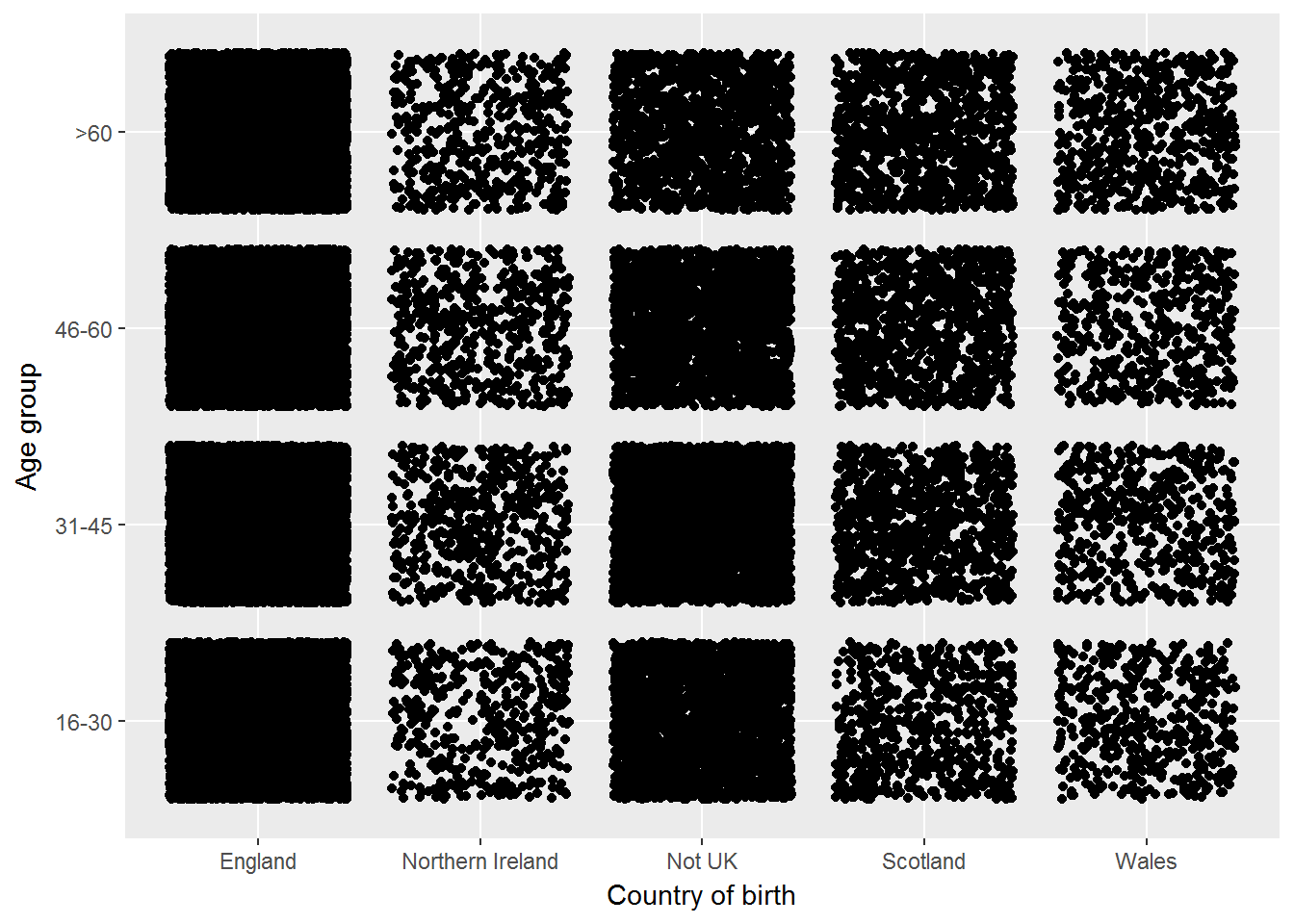## 7.7 Showing the relationships by group

Exercise. Use facets to visualise the association between age and BMI by country of birth.

W1 %>%
filter(!is.na(cbirth)) %>%
ggplot(aes(x = a_dvage, y= bmi)) +
geom_point() +
geom_smooth() +
facet_wrap(~ cbirth)